# design of voltmeter

most meter movements are sensitive devices. Some D’Arsonval movements have full-scale deflection current ratings as little as 50 µA, with an (internal) wire resistance of less than 1000 Ω. This makes for a voltmeter with a full-scale rating of only 50 millivolts (50 µA X 1000 Ω)! In order to build voltmeters with practical (higher voltage) scales from such sensitive movements, we need to find some way to reduce the measured quantity of voltage down to a level the movement can handle.

Let’s start our example problems with a D’Arsonval meter movement having a full-scale deflection rating of 1 mA and a coil resistance of 500 Ω: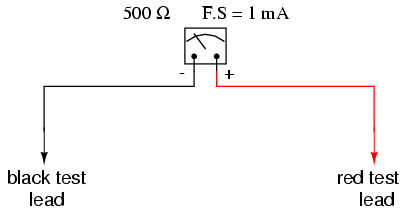Using Ohm’s Law (E=IR), we can determine how much voltage will drive this meter movement directly to full scale:

E = I R

E = (1 mA)(500 Ω)

E = 0.5 volts

If all we wanted was a meter that could measure 1/2 of a volt, the bare meter movement we have here would suffice. But to measure greater levels of voltage, something more is needed. To get an effective voltmeter meter range in excess of 1/2 volt, we’ll need to dsign a circuit allowing only a precise proportion of measured voltage to drop across the meter movement. This will extend the meter movement’s range to higher voltages. Correspondingly, we will need to re-label the scale on the meter face to indicate its new measurement range with this proportioning circuit connected.

But how do we create the necessary proportioning circuit? Well, if our intention is to allow this meter movement to measure a greater voltage than it does now, what we need is a voltage divider circuit to proportion the total measured voltage into a lesser fraction across the meter movement’s connection points. Knowing that voltage divider circuits are built from series resistances, we’ll connect a resistor in series with the meter movement (using the movement’s own internal resistance as the second resistance in the divider):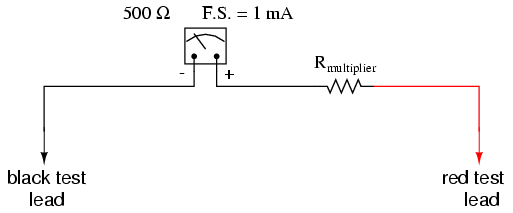The series resistor is called a “multiplier” resistor because it multiplies the working range of the meter movement as it proportionately divides the measured voltage across it. Determining the required multiplier resistance value is an easy task if you’re familiar with series circuit analysis.

For example, let’s determine the necessary multiplier value to make this 1 mA, 500 Ω movement read exactly full-scale at an applied voltage of 10 volts. To do this, we first need to set up an E/I/R table for the two series components: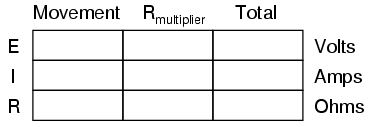Knowing that the movement will be at full-scale with 1 mA of current going through it, and that we want this to happen at an applied (total series circuit) voltage of 10 volts, we can fill in the table as such:There are a couple of ways to determine the resistance value of the multiplier. One way is to determine total circuit resistance using Ohm’s Law in the “total” column (R=E/I), then subtract the 500 Ω of the movement to arrive at the value for the multiplier:Another way to figure the same value of resistance would be to determine voltage drop across the movement at full-scale deflection (E=IR), then subtract that voltage drop from the total to arrive at the voltage across the multiplier resistor. Finally, Ohm’s Law could be used again to determine resistance (R=E/I) for the multiplier:Either way provides the same answer (9.5 kΩ), and one method could be used as verification for the other, to check accuracy of work.With exactly 10 volts applied between the meter test leads (from some battery or precision power supply), there will be exactly 1 mA of current through the meter movement, as restricted by the “multiplier” resistor and the movement’s own internal resistance. Exactly 1/2 volt will be dropped across the resistance of the movement’s wire coil, and the needle will be pointing precisely at full-scale. Having re-labeled the scale to read from 0 to 10 V (instead of 0 to 1 mA), anyone viewing the scale will interpret its indication as ten volts. Please take note that the meter user does not have to be aware at all that the movement itself is actually measuring just a fraction of that ten volts from the external source. All that matters to the user is that the circuit as a whole functions to accurately display the total, applied voltage.

This is how practical electrical meters are designed and used: a sensitive meter movement is built to operate with as little voltage and current as possible for maximum sensitivity, then it is “fooled” by some sort of divider circuit built of precision resistors so that it indicates full-scale when a much larger voltage or current is impressed on the circuit as a whole. We have examined the design of a simple voltmeter here. Ammeters follow the same general rule, except that parallel-connected “shunt” resistors are used to create a current divider circuit as oposed to the series-connected voltage divider “multiplier” resistors used for voltmeter designs.

Generally, it is useful to have multiple ranges established for an electromechanical meter such as this, allowing it to read a broad range of voltages with a single movement mechanism. This is accomplished through the use of a multi-pole switch and several multiplier resistors, each one sized for a particular voltage range: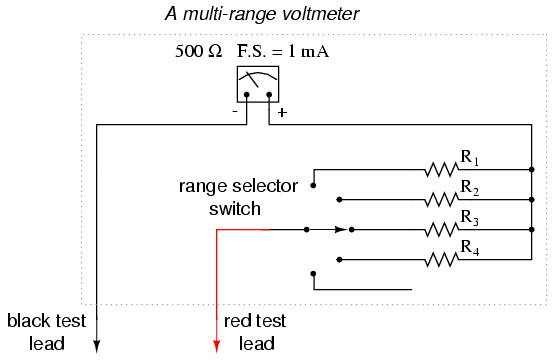The five-position switch makes contact with only one resistor at a time. In the bottom (full clockwise) position, it makes contact with no resistor at all, providing an “off” setting. Each resistor is sized to provide a particular full-scale range for the voltmeter, all based on the particular rating of the meter movement (1 mA, 500 Ω). The end result is a voltmeter with four different full-scale ranges of measurement. Of course, in order to make this work sensibly, the meter movement’s scale must be equipped with labels appropriate for each range.

With such a meter design, each resistor value is determined by the same technique, using a known total voltage, movement full-scale deflection rating, and movement resistance. For a voltmeter with ranges of 1 volt, 10 volts, 100 volts, and 1000 volts, the multiplier resistances would be as follows: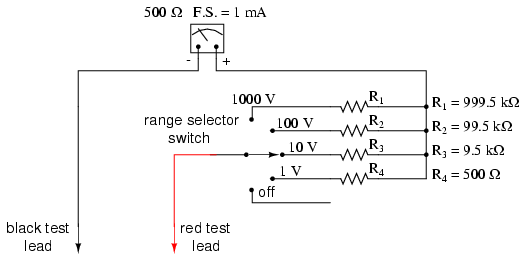Note the multiplier resistor values used for these ranges, and how odd they are. It is highly unlikely that a 999.5 kΩ precision resistor will ever be found in a parts bin, so voltmeter designers often opt for a variation of the above design which uses more common resistor values:With each successively higher voltage range, more multiplier resistors are pressed into service by the selector switch, making their series resistances add for the necessary total. For example, with the range selector switch set to the 1000 volt position, we need a total multiplier resistance value of 999.5 kΩ. With this meter design, that’s exactly what we’ll get:

RTotal = R4 + R3 + R2 + R1

RTotal = 900 kΩ + 90 kΩ + 9 kΩ + 500 Ω

RTotal = 999.5 kΩ

The advantage, of course, is that the individual multiplier resistor values are more common (900k, 90k, 9k) than some of the odd values in the first design (999.5k, 99.5k, 9.5k). From the perspective of the meter user, however, there will be no discernible difference in function.

REVIEW:
Extended voltmeter ranges are created for sensitive meter movements by adding series “multiplier” resistors to the movement circuit, providing a precise voltage division ratio.

## Voltmeter impact on measured circuit

Every meter impacts the circuit it is measuring to some extent, just as any tire-pressure gauge changes the measured tire pressure slightly as some air is let out to operate the gauge. While some impact is inevitable, it can be minimized through good meter design.

Since voltmeters are always connected in parallel with the component or components under test, any current through the voltmeter will contribute to the overall current in the tested circuit, potentially affecting the voltage being measured. A perfect voltmeter has infinite resistance, so that it draws no current from the circuit under test. However, perfect voltmeters only exist in the pages of textbooks, not in real life! Take the following voltage divider circuit as an extreme example of how a realistic voltmeter might impact the circuit its measuring:With no voltmeter connected to the circuit, there should be exactly 12 volts across each 250 MΩ resistor in the series circuit, the two equal-value resistors dividing the total voltage (24 volts) exactly in half. However, if the voltmeter in question has a lead-to-lead resistanc of 10 MΩ (a common amount for a modern digital voltmeter), its resistance will create a parallel subcircuit with the lower resistor of the divider when connected: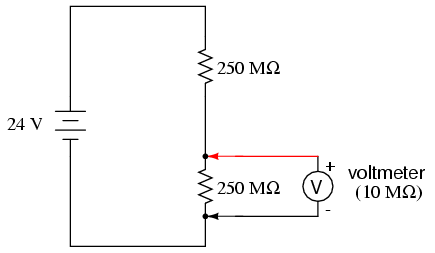This effectively reduces the lower resistance from 250 MΩ to 9.615 MΩ (250 MΩ and 10 MΩ in parallel), drastically altering voltage drops in the circuit. The lower resistor will now have far less voltage across it than before, and the upper resistor far more.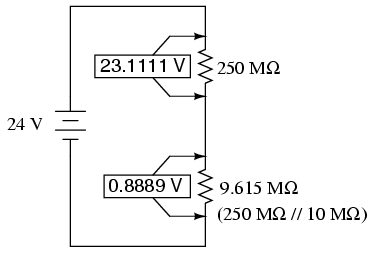A voltage divider with resistance values of 250 MΩ and 9.615 MΩ will divide 24 volts into portions of 23.1111 volts and 0.8889 volts, respectively. Since the voltmeter is part of that 9.615 MΩ resistance, that is what it will indicate: 0.8889 volts.

Now, the voltmeter can only indicate the voltage its connected across. It has no way of “knowing” there was a potential of 12 volts dropped across the lower 250 MΩ resistor before it was connected across it. The very act of connecting the voltmeter to the circuit makes it part of the circuit, and the voltmeter’s own resistance alters the resistance ratio of the voltage divider circuit, consequently affecting the voltage being measured.

Imagine using a tire pressure gauge that took so great a volume of air to operate that it would deflate any tire it was connected to. The amount of air consumed by the pressure gauge in the act of measurement is analogous to the current taken by the voltmeter movement to move the needle. The less air a pressure gauge requires to operate, the less it will deflate the tire under test. The less current drawn by a voltmeter to actuate the needle, the less it will burden the circuit under test.

This effect is called loading, and it is present to some degree in every instance of voltmeter usage. The scenario shown here is worst-case, with a voltmeter resistance substantially lower than the resistances of the divider resistors. But there always will be some degree of loading, causing the meter to indicate less than the true voltage with no meter connected. Obviously, the higher the voltmeter resistance, the less loading of the circuit under test, and that is why an ideal voltmeter has infinite internal resistance.

Voltmeters with electromechanical movements are typically given ratings in “ohms per volt” of range to designate the amount of circuit impact created by the current draw of the movement. Because such meters rely on different values of multiplier resistors to give different measurement ranges, their lead-to-lead resistances will change depending on what range they’re set to. Digital voltmeters, on the other hand, often exhibit a constant resistance across their test leads regardless of range setting (but not always!), and as such are usually rated simply in ohms of input resistance, rather than “ohms per volt” sensitivity.

What “ohms per volt” means is how many ohms of lead-to-lead resistance for every volt of range setting on the selector switch. Let’s take our example voltmeter from the last section as an example:On the 1000 volt scale, the total resistance is 1 MΩ (999.5 kΩ + 500Ω), giving 1,000,000 Ω per 1000 volts of range, or 1000 ohms per volt (1 kΩ/V). This ohms-per-volt “sensitivity” rating remains constant for any range of this meter: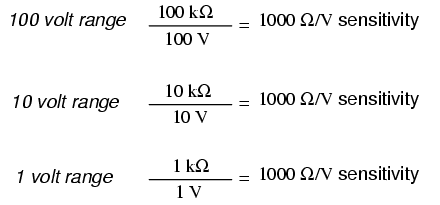The astute observer will notice that the ohms-per-volt rating of any meter is determined by a single factor: the full-scale current of the movement, in this case 1 mA. “Ohms per volt” is the mathematical reciprocal of “volts per ohm,” which is defined by Ohm’s Law as current (I=E/R). Consequently, the full-scale current of the movement dictates the Ω/volt sensitivity of the meter, regardless of what ranges the designer equips it with through multiplier resistors. In this case, the meter movement’s full-scale current rating of 1 mA gives it a vltmeter sensitivity of 1000 Ω/V regardless of how we range it with multiplier resistors.

To minimize the loading of a voltmeter on any circuit, the designer must seek to minimize the current draw of its movement. This can be accomplished by re-designing the movement itself for maximum sensitivity (less current required for full-scale deflection), but the tradeoff here is typically ruggedness: a more sensitive movement tends to be more fragile.

Another approach is to electronically boost the current sent to the movement, so that very little current needs to be drawn from the circuit under test. This special electronic circuit is known as an amplifier, and the voltmeter thus constructed is an amplified voltmeter.The internal workings of an amplifier are too complex to be discussed at this point, but suffice it to say that the circuit allows the measured voltage to control how much battery current is sent to the meter movement. Thus, the movement’s current needs are supplied by a battery internal to the voltmeter and not by the circuit under test. The amplifier still loads the circuit under test to some degree, but generally hundreds or thousands of times less than the meter movement would by itself.

Before the advent of semiconductors known as “field-effect transistors,” vacuum tubes were used as amplifying devices to perform this boosting. Such vacuum-tube voltmeters, or (VTVM’s) were once very popular instruments for electronic test and measurement. Here is a photograph of a very old VTVM, with the vacuum tube exposed!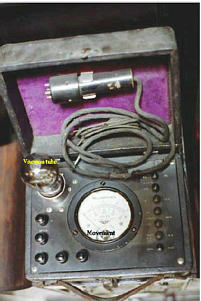width=”201″ height=”301″/>

Now, solid-state transistor amplifier circuits accomplish the same task in digital meter designs. While this approach (of using an amplifier to boost the measured signal current) works well, it vastly complicates the design of the meter, making it nearly impossible for the beginning electronics student to comprehend its internal workings.

A final, and ingenious, solution to the problem of voltmeter loading is that of the potentiometric or null-balance instrument. It requires no advanced (electronic) circuitry or sensitive devices like transistors or vacuum tubes, but it does require greater technician involvement and skill. In a potentiometric instrument, a precision adjustable voltage source is compared against the measured voltage, and a sensitive device called a null detector is used to indicate when the two voltages are equal. In some circuit designs, a precision potentiometer is used to provide the adjustable voltage, hence the label potentiometric. When the voltages are equal, there will be zero current drawn from the circuit under test, and thus the measured voltage should be unaffected. It is easy to show how this works with our last example, the high-resistance voltage divider circuit:The “null detector” is a sensitive device capable of indicating the presence of very small voltages. If an electromechanical meter movement is used as the null detector, it will have a spring-centered needle that can deflect in either direction so as to be useful for indicating a voltage of either polarity. As the purpose of a null detector is to accurately indicate a condition of zero voltage, rather than to indicate any specific (nonzero) quantity as a normal voltmeter would, the scale of the instrument used is irrelevant. Null detectors are typically designed to be as sensitive as possible in order to more precisely indicate a “null” or “balance” (zero voltage) condition.

An extremely simple type of null detector is a set of audio headphones, the speakers within acting as a kind of meter movement. When a DC voltage is initially applied to a speaker, the resulting current through it will move the speaker cone and produce an audible “click.” Another “click” sound will be heard when the DC source is isconnected. Building on this principle, a sensitive null detector may be made from nothing more than headphones and a momentary contact switch:If a set of “8 ohm” headphones are used for this purpose, its sensitivity may be greatly increased by connecting it to a device called a transformer. The transformer exploits principles of electromagnetism to “transform” the voltage and current levels of electrical energy pulses. In this case, the type of transformer used is a step-down transformer, and it converts low-current pulses (created by closing and opening the pushbutton switch while connected to a small voltage source) into higher-current pulses to more efficiently drive the speaker cones inside the headphones. An “audio output” transformer with an impedance ratio of 1000:8 is ideal for this purpose. The transformer also increases detector sensitivity by accumulating the energy of a low-current signal in a magnetic field for sudden release into the headphone speakers when the switch is opened. Thus, it will produce louder “clicks” for detecting smaller signals:Connected to the potentiometric circuit as a null detector, the switch/transformer/headphone arrangement is used as such:The purpose of any null detector is to act like a laboratory balance scale, indicating when the two voltages are equal (absence of voltage between points 1 and 2) and nothing more. The laboratory scale balance beam doesn’t actually weigh anything; rather, it simply indicates equality between the unknown mass and the pile of standard (calibrated) masses.Likewise, the null detector simply indicates when the voltage between points 1 and 2 are equal, which (according to Kirchhoff’s Voltage Law) will be when the adjustable voltage source (the battery symbol with a diagonal arrow going through it) is precisely equal in voltage to the drop across R2.

To operate this instrument, the technician would manually adjust the output of the precision voltage source until the null detector indicated exactly zero (if using audio headphones as the null detector, the technician would repeatedly press and release the pushbutton switch, listening for silence to indicate that the circuit was “balanced”), and then note the source voltage as indicated by a voltmeter connected across the precision voltage source, that indication being representative of the voltage across the lower 250 MΩ resistor:The voltmeter used to directly measure the precision source need not have an extremely high Ω/V sensitivity, because the source will supply all the current it needs to operate. So long as there is zero voltage across the null detector, there will be zero current between points 1 and 2, equating to no loading of the divider circuit under test.

It is worthy to reiterate the fact that this method, properly executed, places almost zero load upon the measured circuit. Ideally, it places absolutely no load on the tested circuit, but to achieve this ideal goal the null detector would have to have absolutely zero voltage across it, which would require an infinitely sensitive null meter and a perfect balance of voltage from the adjustable voltage source. However, despite its practical inability to achieve absolute zero loading, a potentiometric circuit is still an excellent technique for measuring voltage in high-resistance circuits. And unlike the electronic amplifier solution, which solves the problem with advanced technology, the potentiometric method achieves a hypothetically perfect solution by exploiting a fundamental law of electricity (KVL).

REVIEW:
An ideal voltmeter has infinite resistance.
Too low of an internal resistance in a voltmeter will adversely affect the circuit being measured.
Vacuum tube voltmeters (VTVM’s), transistor voltmeters, nd potentiometric circuits are all means of minimizing the load placed on a measured circuit. Of these methods, the potentiometric (“null-balance”) technique is the only one capable of placing zero load on the circuit.
A null detector is a device built for maximum sensitivity to small voltages or currents. It is used in potentiometric voltmeter circuits to indicate the absence of voltage between two points, thus indicating a condition of balance between an adjustable voltage source and the voltage being measured.

Related

COMMENT songs